# NEAT AND NES ALGORITHMS

The South Korean Ministry of the Interior provides a cryptography library for its GPKI program. This library contains two symmetric encryption algorithms, NEAT and NES, which have not been published. We reverse engineered these algorithms, and are providing descriptions and working implementations.

(Korean version of this post can be found here.)

## NEAT

National Encryption Algorithm (NEAT) is a block cipher with a 128-bit block size and 128-bit key size. It is believed to have been developed in 1997 by KISA. It takes inspiration from the IDEA and RC5 block ciphers. It operates on the two 64-bit halves of the block, and combines portions of the two halves with a bitwise XOR.

### Operation

NEAT has 12 full rounds and one half round (no mix). Each round splits the block into 16-bit integers and performs several operations on these integers. Similar to IDEA, NEAT interleaves operations from different groups:

• Bitwise XOR (⊕)
• Multiplication modulo 216+1, with 0x0000 treated as 216 (⊙)

NEAT borrows an addtional operation, data dependent rotation, from RC5:

• Rotation (⋘)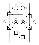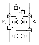The 128-bit block is split into two 64-bit halves. The left half is fed into F and the right half is fed into F-1. The round functions split each 64-bit half into four 16-bit integers, perform operations, and then concatenate back into a 64-bit block. Each round splits the 64-bit round key into four 16-bit subkeys, Kn, which are used by the multiplication operation.

The MIX function does a bitwise XOR of some bits from the left half and the right half. The set of bits mixed together depend on the round key.

The last round is a half round without the MIX function. Instead, the left halves and right halves are swapped. This is important because it allows us to use the same code for encryption and decryption.

### Key Schedule

The NEAT algorithm uses 13 round keys, one per full round and one for the last round. These round keys are generated using the same algorithm as encryption. The provided master key is treated as a 128-bit data block, and the round key is fixed:

Each round will generate two 64-bit round keys, so after 6.5 rounds we will have enough round keys. The mix function for each round is determined by K1 ^ K4 mod 128.

#### Decryption

As mentioned previously, we can use the same code for encryption and decryption. The only difference is that after generating the round keys for encryption we need to transform them into decryption round keys.

Since F and F-1 use different Kn we need to swap (K1, K2) with (K3, K4). Also, we need to reverse the order of the round keys so that we use the round keys from the last round (encryption) during the first round (decryption). Lastly, in order to reverse the multiplication operation we need to calculate the multiplicative inverse of each subkey.

## NES

National Encryption Standard (NES) is a block cipher with a 256-bit block size and 256-bit key size, developed in 2003. Similar to AES, it is a substitution-permutation network (SPN) and operates on each 8-bit byte of the block. It appears to be inspired by Anubis and KHAZAD, which are involutional SPN ciphers. It also has some similarities to the publically released ARIA block cipher.

### Operation

NES operates on each byte as an element in Rijndael’s finite field. Bitwise XOR is used as the addition operator, and multiplication is modulo the irreducibble polynomial . It uses a S-box for substitution and the permutation can be viewed as a 8x8 matrix multiplication.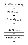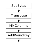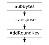NES has 11 full rounds and a last round without permutation. Each round adds a 128-bit round key to the state. Before the first round, an initial round key is added to the initial state. Depending on whether the full round is even or odd, it will use different matrix for permutation.

1. Initial Round (Round 0)
2. Rounds (Round 1~11)
1. Substitution
2. Transposition
3. MixColumns (permutation)
3. Final Round
1. Substitution
2. Transposition

Assuming an input state of the form , the substitution and transposition operations can be viewed as matrix operations resulting in a 8x4 matrix. The function S applies substitution on a byte using the S-box.

In a full round, the resultant matrix can be transformed by matrix multiplication with one of the two following matrices. In a half round, no permutation is performed, which is the same as multiplication with the identity matrix.

or

In either case, the resultant matrix is stored as and is bitwise XOR with the round key.

### Key Schedule

The key schedule is based on the round function used in decryption, using the inverse S-box and one of the inverse P-box (i.e. no concept of even/odd rounds). The state is initialized with the supplied 256-bit master key. The state is viewed as eight 32-bit integers, b0b7.

Each key schedule round operates (substitution, permutation, and add round key) on 32 bits of the state, bj, with bj+1 as the round key. The 32-bit output of each key schedule round, b’j, is bitwise XOR with other bits in the state to generate the full 128-bit encryption round key: [ b’j, b’j ^ bj+2, b’j ^ bj+3, b’j ^ bj+4].

#### Decryption

NES is an almost involutional SPN block cipher. Encryption and decryption share the same code and differ only in the key schedule, S-box, and P-box. During decryption, the following inverse matrices are used for the even and odd rounds:

The decryption key schedule can be generated from the encryption key schedule by first reversing the order of the round keys. Then, for each full round key, apply the appropriate inverse matrix to the round key. This effectively swaps the order of the AddRoundKey and MixColumns steps in the round function.

## Implementations

On the GitHub repository, you can find working C and Python implementations of both NEAT and NES. Since these algorithms have not been formally published by their authors, it is unknown if they are covered by any patents. The implementations have been tested against the library we used as a reference.기업문화 엿볼 때, 더팀스

기업문화 엿볼 때, 더팀스